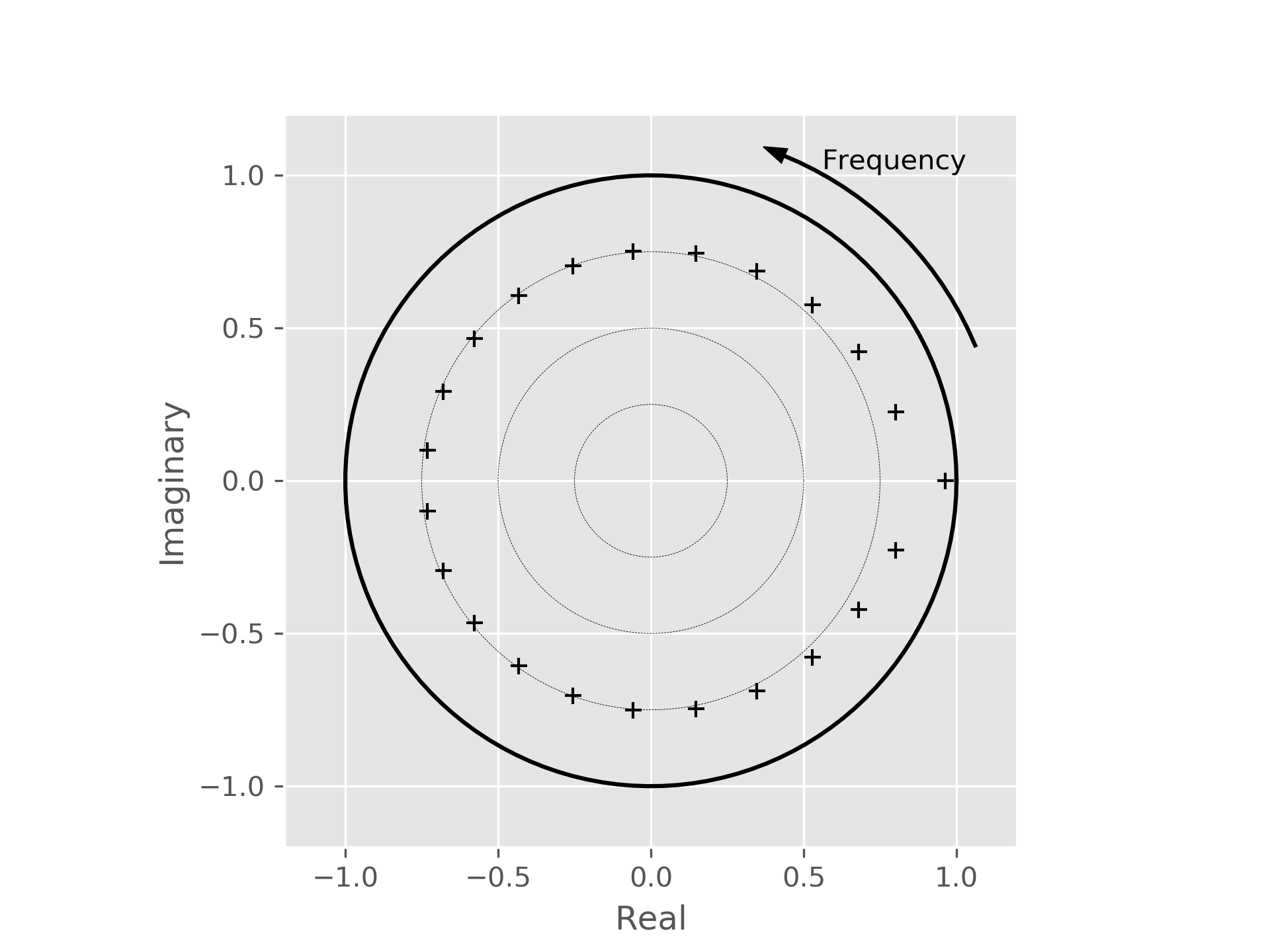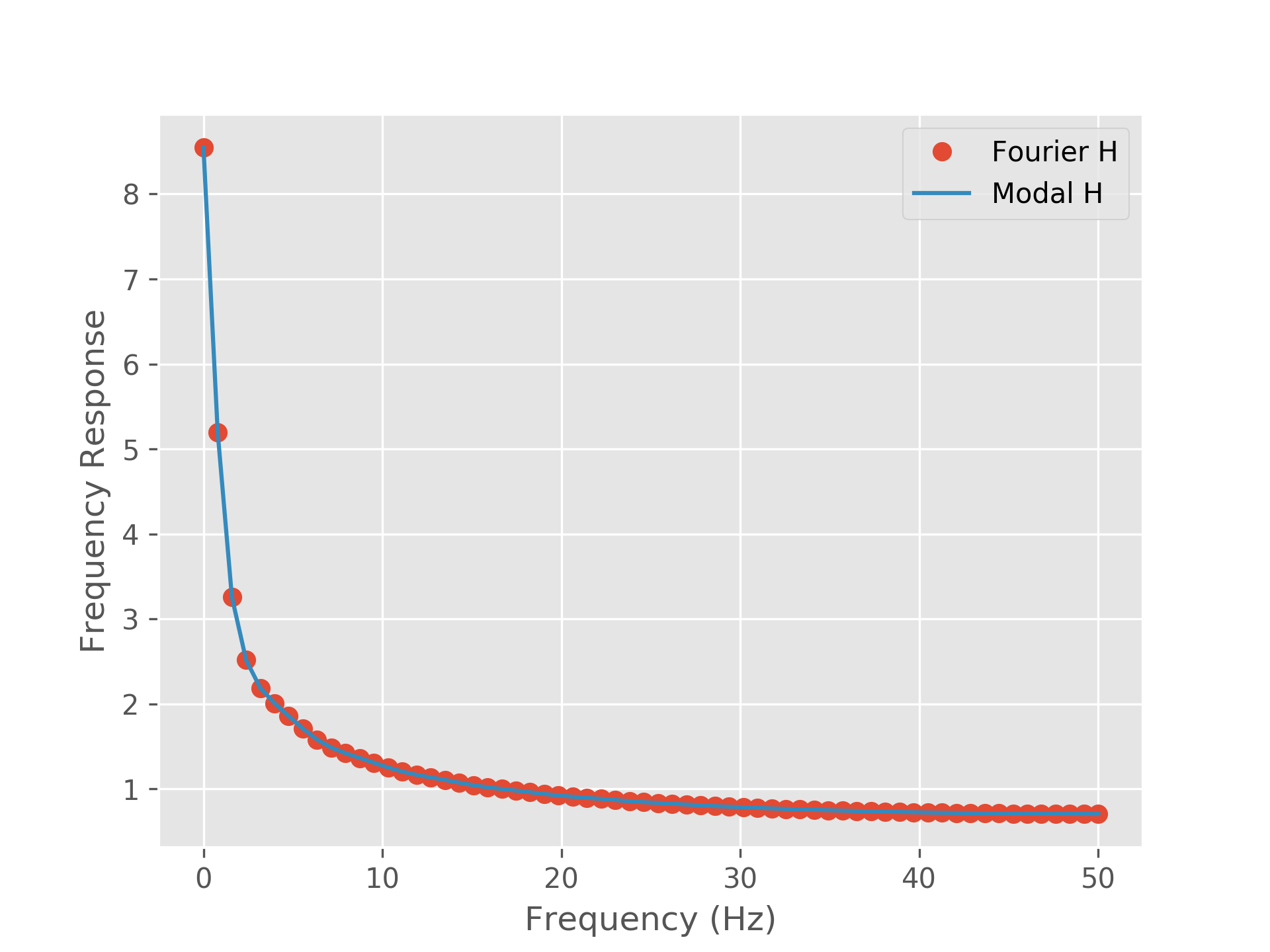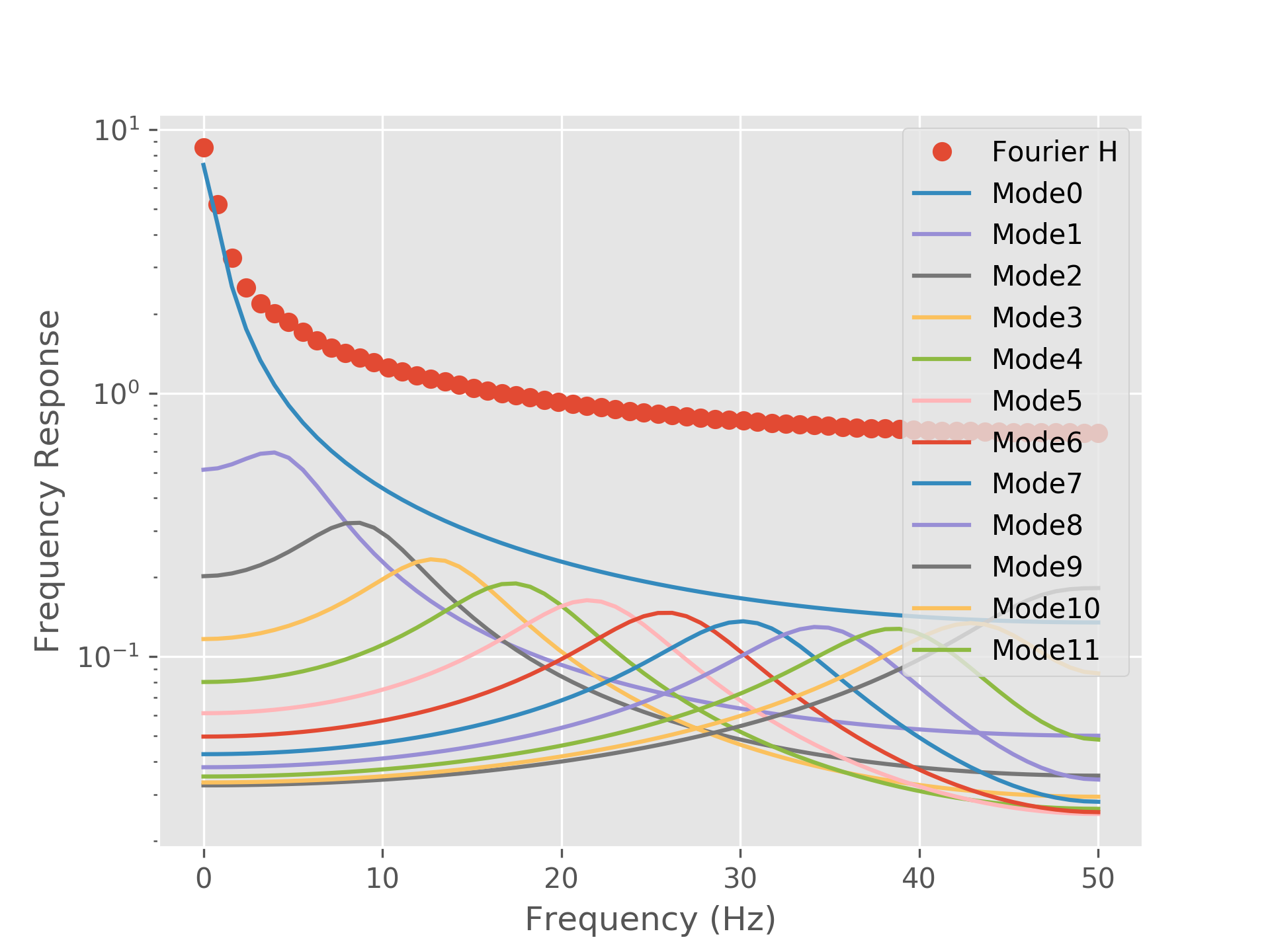# Tutorial 1 - A pink noise system¶

In this tutorial we will demonstrate how to set up a simple univariate AR model which models a pink noise process. We will use the model to demonstrate how to extract the transfer function using both a Fourier and Modal estimator.

We start by importing the routines we will need from the numpy, sails and matplotlib. We set the ggplot style for all plots in the tutorials.

import numpy as np
from sails import generate_pink_roots, root_plot, model_from_roots
import matplotlib.pyplot as plt

plt.style.use('ggplot')


We now define the meta-parameters of our system. We will arbitrarily pretend that we are using a sampling rate of 100Hz. From this, we can compute our Nyquist frequency, and a vector of frequencies at which we will evaluate measures such as our Fourier estimator. The variable freq_vect will come up often during our tutorials. In this case, we will estimate 64 frequencies which are linearly spaced between 0Hz and Nyquist. You can alter freq_vect and examine how this affects the results of the later estimations.

sample_rate = 100
nyq = sample_rate / 2.

freq_vect = np.linspace(0, nyq, 64)


As mentioned above, for our first system we will emulate a system which behaves as a pink noise process. Whereas we would normally learn our model from some data, for this example we will construct a model from the polynomial form of the AR model. A function (generate_pink_roots()) has been provided which calculates a set of roots which will generate the appropriate roots:

roots = generate_pink_roots(1)


Given the roots of the model, we can plot these in a Z-plane representation to examine them. A function (root_plot()) is provided to make this straightforward.

ax = root_plot(roots)
ax.figure.show()The plot shows frequency increasing counterclockwise from the x-axis. Nyquist frequency is on the negative x-axis. For real systems, the structure of the pole plot will be mirrored across the x-axis.

As mentioned above, we would normally set up a model by learning the parameters from data. During this exploratory tutorial, we can analytically create the model from the roots. The model_from_roots() function will perform this task for you.

m = model_from_roots(roots)


No matter how we create our model, it will be a subclass of AbstractLinearModel. All subclasses of AbstractLinearModel provide a number of guarantees so that they can all be used with the various estimator routines we discuss below. We will discuss fitting a model from data in a later tutorial.

Now that we have our model, we can extract the transfer function (H) using two different methods. The first method extracts the transfer function using a Fourier transform of the parameters.

from sails import FourierMvarMetrics

F = FourierMvarMetrics.initialise(m, sample_rate, freq_vect)
F_H = F.H


The second method using a modal decomposition of the parameter matrix to break the parameters apart into, hopefully, interpretable components. We first of all create a ModalMvarMetrics and then use the MvarModalDecomposition object inside of this to extract the transfer function for each mode. Each mode will consist of either a pair of poles in the complex plane or a single real mode. The per_mode_transfer_function() will take this into account for you.

from sails import ModalMvarMetrics

M = ModalMvarMetrics.initialise(m, sample_rate, freq_vect)
M_H = M.modes.per_mode_transfer_function(sample_rate, freq_vect)


We can plot our modes in both forms to examine the relationship between them. Firstly, we sum the modal transfer function across all modes to recover the Fourier based transfer function (to within computational precision).

fourier_H = np.abs(F_H).squeeze()
modal_H = np.abs(M_H.sum(axis=1)).squeeze()

# Check the two forms are equivalent
equiv = np.allclose( fourier_H, modal_H )
ssdiff = np.sum( (fourier_H - modal_H)**2 )
print('Fourier and Modal transfer functions equivalent: {0}'.format(equiv))
print('Sum-square residual difference: {0}'.format(ssdiff))

# Plot our fourier and modal spectra

f2 = plt.figure()

plt.plot(freq_vect, fourier_H, 'o');
plt.plot(freq_vect, modal_H);

plt.xlabel('Frequency (Hz)')
plt.ylabel('Frequency Response')

plt.legend(['Fourier H', 'Modal H'])

plt.savefig('tutorial1_2.png', dpi=300)
f2.show()Finally, we can see how each mode contributes to the overall transfer function shape by plotting each mode separately. Each mode contributes a single uni-modal resonance to the spectrum. In this case there are no clear peaks in the spectrum, just the 1/f shape - as such, all the mode peaks sum together to make a smooth spectrum. Note that we’re plotting the modes on a log y-scale as some modes make a very small contribution to the overall transfer function.

fourier_H = np.abs(F_H).squeeze()
modal_H = np.abs(M_H).squeeze()

# Plot our fourier and modal spectra

f2 = plt.figure()

plt.semilogy(freq_vect, fourier_H, 'o');
plt.semilogy(freq_vect, modal_H);

plt.xlabel('Frequency (Hz)')
plt.ylabel('Frequency Response')

legend = ['Mode' + str(ii) for ii in range(modal_H.shape)]
plt.legend(['Fourier H'] + legend)

plt.savefig('tutorial1_3.png', dpi=300)
f2.show()